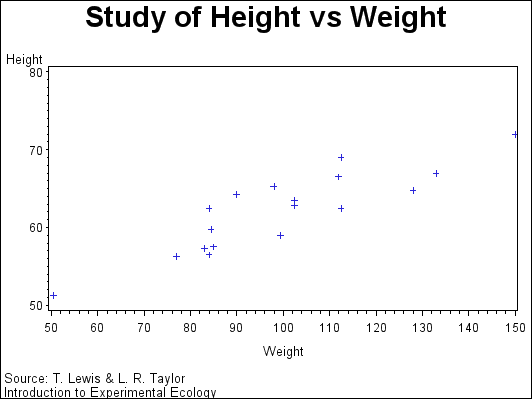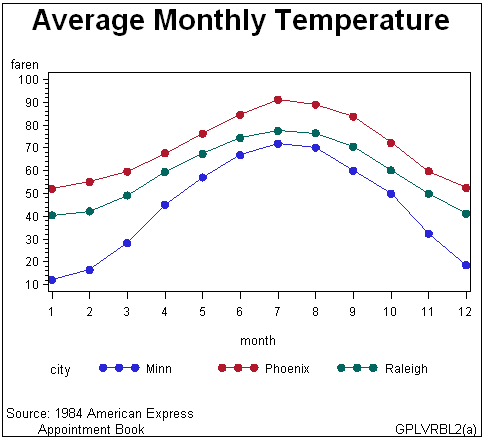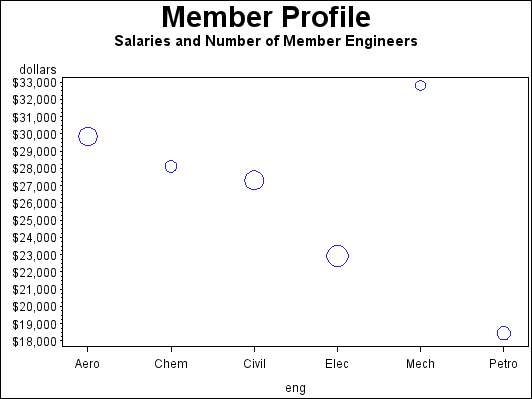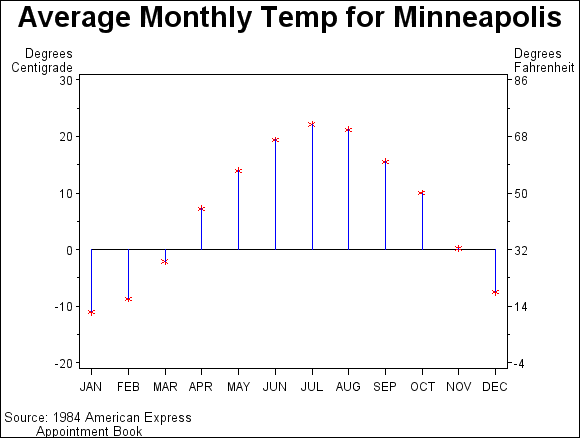The GPLOT Procedure

# Overview

The GPLOT procedure plots the values of two or more variables on a set of coordinate axes (X and Y). The coordinates of each point on the plot correspond to two variable values in an observation of the input data set. The procedure can also generate a separate plot for each value of a third (classification) variable. It can also generate bubble plots in which circles of varying proportions representing the values of a third variable are drawn at the data points.

The procedure produces a variety of two-dimensional graphs including the following plots:

• simple scatter plots

• overlay plots in which multiple sets of data points display on one set of axes

• plots against a second vertical axis

• bubble plots

• logarithmic plots (controlled by the AXIS statement)

In conjunction with the SYMBOL statement, the GPLOT procedure can produce join plots, high-low charts, needle plots, and plots with simple or spline-interpolated lines. The SYMBOL statement can also display regression lines on scatter plots.

The GPLOT procedure is useful for the following tasks:

• displaying a long series of data, showing trends and patterns

• interpolating between data points

• extrapolating beyond existing data with the display of regression lines and confidence limits.

Plots of two variables display the values of two variables as data points on one horizontal axis (X) and one vertical axis (Y). Each pair of X and Y values forms a data point.

The following figure shows a simple scatter plot that plots the values of the variable HEIGHT on the vertical axis and the variable WEIGHT on the horizontal axis. By default, the PLOT statement scales the axes to include the maximum and minimum data values and displays a symbol at each data point. It labels each axis with the name of its variable or an associated label and displays the value of each major tick mark.The program for this plot is in Plotting Two Variables. For more information on producing scatter plots, see PLOT Statement.

You can also overlay two or more plots (multiple sets of data points) on a single set of axes and you can apply a variety of interpolation techniques to these plots. See About Interpolation Methods.

Plots that use a classification variable produce a separate set of data points for each unique value of the classification variable and display all sets of data points on one set of axes.

The following figure shows multiple line plots that compare yearly temperature trends for three cities. The legend explains the values of the classification variable, CITY.By default, plots with a classification variable generate a legend. In the code that generates the plot for Plotting Three Variables, a SYMBOL statement connects the data points and specifies the plot symbol that is used for each value of the classification variable (CITY). For more information on how to produce plots with a classification variable, see PLOT Statement.

Bubble plots represent the values of three variables by drawing circles of varying sizes at points that are plotted on the vertical and horizontal axes. Two of the variables determine the location of the data points, while the values of the third variable control the size of the circles.

Bubble Plot (GPLBUBL1) shows a bubble plot in which each bubble represents a category of engineer that is shown on the horizontal axis. The location of each bubble in relation to the vertical axis is determined by the average salary for the category. The size of each bubble represents the number of engineers in the category relative to the total number of engineers in the data.

By default, the BUBBLE statement scales the axes to include the maximum and minimum data values and draws a circle at each data point. It labels each axis with the name of its variable or an associated label and displays the value of each major tick mark.The program for this plot is in Generating a Simple Bubble Plot. For more information on producing bubble plots, see BUBBLE Statement.

Plots with two vertical axes have a right vertical axis that can do the following:

• display the same variable values as the left axis

• display left axis values in a different scale

• plot a second response (Y) variable, thereby producing one or more overlay plots

In the following figure, the right axis displays the values of the vertical coordinates in a different scale from the scale that is used for the left axis.The program for this plot is in Plotting with Different Scales of Values. For more information on how to produce plots with a right vertical axis, see PLOT2 Statement and BUBBLE2 Statement.

In addition to these graphs, you can produce other types of plots such as box plots or high-low-close charts by specifying various interpolation methods with the SYMBOL statement. Use the SYMBOL statement to do the following tasks:

• connect the data points with straight lines

• specify regression analysis to fit a line to the points and can display lines for confidence limits

• connect the data points to the zero line on the vertical axis

• display the minimum and maximum values of Y at each X value and mark the mean value, display standard deviations that connect the data points with lines or bars, generate box plots, or plot high-low-close stock market data

• specify that a pattern fills the polygon that is defined by data points

• smooth plot lines with spline interpolation

• use a step function to connect the data points

SYMBOL Statement describes all interpolation methods.Previous Page | Next Page | Top of Page